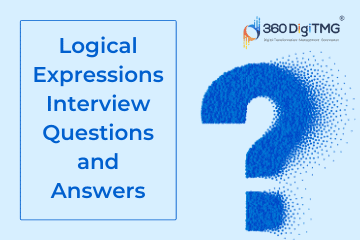Home / Blog / Interview Questions on Data Science / Logical Expressions Interview Questions and Answers

# Logical Expressions Interview Questions and Answers

• September 06, 2022
• 5807
• 44### Meet the Author : Mr. Bharani Kumar

Bharani Kumar Depuru is a well known IT personality from Hyderabad. He is the Founder and Director of Innodatatics Pvt Ltd and 360DigiTMG. Bharani Kumar is an IIT and ISB alumni with more than 17 years of experience, he held prominent positions in the IT elites like HSBC, ITC Infotech, Infosys, and Deloitte. He is a prevalent IT consultant specializing in Industrial Revolution 4.0 implementation, Data Analytics practice setup, Artificial Intelligence, Big Data Analytics, Industrial IoT, Business Intelligence and Business Management. Bharani Kumar is also the chief trainer at 360DigiTMG with more than Ten years of experience and has been making the IT transition journey easy for his students. 360DigiTMG is at the forefront of delivering quality education, thereby bridging the gap between academia and industry.

• ### Logistic regression method is _________________.

• a) Any type of output variable.
• b) Only for binary classification output.
• c) More than two outcomes.
• d) None of the above.

Answer - b) Only for binary classification output

• ### Mathematical understanding of the _________________and the natural logarithm function is used to understand what logistic regression is and how it works.

• a) Sigmoid function.
• b) Linear function.
• c) Tanh function.
• d) Relu function.

• ### The sigmoid function has values very close to either ____________across most of its domain.

• a) Infinity.
• b) Negative values.
• c) 0 or 1.
• d) Always 0.

Answer - c) 0 or 1

• ### The sigmoid function has values very close to either 0 or 1. This fact makes it suitable for application in ______________ methods.

• a) Classification.
• b) Continuous values prediction.
• c) Both (a) and (b).
• d) None of the above.

• ### In Python, _____________ represent the natural logarithm of x which is used while applying logistic regression.

• a) Only math.log(x).
• b) Only numpy.log(x).
• c) Math.log(x) and numpy.log(x) both.
• d) None of the above.

Answer - c) Math.log(x) and numpy.log(x) both

• ### Logistic regression is a linear classifier, so you’ll use a linear function (x) = b0 + b1x1 + ⋯ + brxr, also called the _____________.

• a) Logit.
• c) Log.
• d) None of the above.

• ### In Logistic regression is a linear classifier, so you’ll use a linear function (x) = b0 + b1x1 + ⋯ + brxr, where the variables b0, b1, …, br are called as _____________

• a) Estimators.
• b) Predicted weights.
• c) Coefficients.
• d) All of the above.

Answer - d) All of the above

• ### The logistic regression function (x) is the sigmoid function of f(x):p(x) =___________________.

• a) 1 / (1 + exp(-f(x)).
• b) (1 + exp(-f(x)).
• c) (1 - exp(-f(x)).
• d) None of the Above.

Answer - a) 1 / (1 + exp((-f(x))

• ### In Logistic regression ,the function p(x) is often interpreted as the predicted probability that the output for a given x is equal to 1. Therefore, 1-(x) is the probability that the output is __________.

• a) 1.
• b) Infinity.
• c) 0.
• d) None of the above.

• ### Logistic regression determines the best predicted weights b0, b1, …, br such that the function p(x) is as close as possible to all actual responses yi, i = 1, …, n, where n is the number of observations. The process of calculating the best weights using available observations is called ___________________.

• a) Data Preprocessing.
• b) Model Validating.
• c) Model training or fitting.
• D) All of the above.

Answer - c) Model training or fitting

• ### In Logistic regression to get the best weights, we usually maximize the log-likelihood function (LLF) for all observations i = 1, …, n. This method is called the ___________________ .

• a) Minimum likelihood estimation.
• b) Maximum likelihood estimation.
• c) True likelihood estimation.
• d) None of the above.

Answer - b) Maximum likelihood estimation

• ### The method maximum likelihood estimation is represented by the equation LLF = _________________ .

• a) Σi(yi log(p(xi)) + (1 - yi) log(1 -p(xi))).
• b) (yi log(p(xi)) + (1 - yi) log(1 -p(xi))).
• c) Σi(yi log(p(xi)) + (1 - yi) log(1 -p(xi))).
• d) None of the above.

Answer - a) Σi(yi log(p(xi)) + (1 - yi) log(1 -p(xi)))

• ### Binary classification has possible types of results, one of which is True negatives which means_________________ .

• a) Correctly predicted negatives (zeros).
• b) Correctly predicted positives (ones).
• c) Incorrectly predicted negatives (zeros).
• d) Incorrectly predicted positives (ones).

Answer - a) Correctly predicted negatives (zeros)

• ### Binary classification has possible types of results, one of which is True positives which means _________________ .

• a) Correctly predicted negatives (zeros).
• b) Correctly predicted positives (ones).
• c) Incorrectly predicted negatives (zeros).
• d) Incorrectly predicted positives (ones).

Answer - b) correctly predicted positives (ones)

• ### Binary classification has possible types of result, one of which is False negatives which means _________________ .

• a) Correctly predicted negatives (zeros).
• b) Correctly predicted positives (ones).
• c) Incorrectly predicted negatives (zeros).
• d) Incorrectly predicted positives (ones).

Answer - c) Incorrectly predicted negatives (zeros)

• ### Binary classification has possible types of result, one of which is False positives which means _________________ .

• a) Correctly predicted negatives (zeros).
• b) Correctly predicted positives (ones).
• c) Incorrectly predicted negatives (zeros).
• d) Incorrectly predicted positives (ones).

Answer - d) Incorrectly predicted positives (ones)

### Data Science Training Institutes in Other Locations